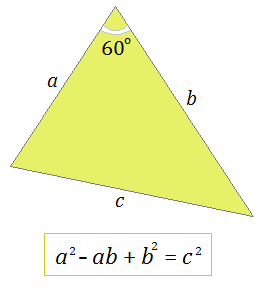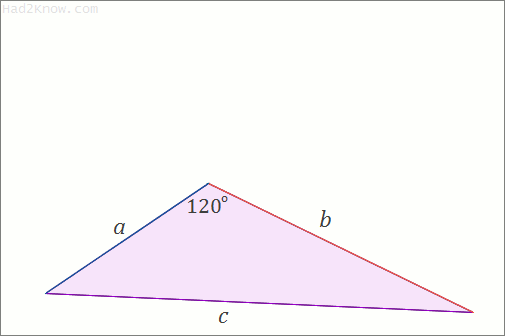# Integer Triangles with a 60° Angle

Just as there are integer right triangles, so there are integer 60° triangles. In such triangles, the side lengths are all integers and one of the angles measures exactly 60°.

If a and b denote the two sides which are adjacent to the 60°, and c denotes the remaining side, then a, b, and c satisfy a Pythagorean-like relation:

a² - ab + b² = c².

This relation holds for all 60° triangles, not just those with integer sides.To find triples (a, b, c) that satisfy the relation a² - ab + b² = c², you can use generator functions similar to the generator functions for Pythagorean triples and 120° triangles, or you can use a trick based on knowledge of 120° triangles. To produce 60° triangles with generator functions, the equations are

a = m² - n²
b = 2mn - n²
c = m² - mn + n²,

where m and n are integers and m > n. This works because

(m² - n²)² - (m² - n²)(2mn - n²) + (2mn - n²)² = (m² - mn + n²)²

### How to Generate 60° Triangles With 120° Triangles

Suppose the triple (x, y, z) represents an integer 120° triangle, i.e., x² + xy + y² = z². Then the triples (x+y, y, z) and (x, x+y, z) represent two integer 60° triangles. To confirm this algebraically, start with the known relation x² + xy + y² = z². Rewrite the left hand side in an equivalent form and simplify:

x² + xy + y² = z²
x² - x² + x² + xy - xy + xy + y² = z²
x² + 2xy + y² - x² - xy + x² = z²
(x+y)² - (x+y)x + x² = z²

This shows that (x, x+y, z) is an integer 60° triangle. A similar argument shows that (x+y, y, z) is as well. For visual proof, see the animated image below:You can use this trick to generate every primitive integer 60° triangle from every primitive integer 120° triangle. The table below shows the first few primitive triples (a, b, c) where c < 100.

 a b c 3 8 7 5 8 7 7 15 13 8 15 13 5 21 19 16 21 19 11 35 31 24 35 31 7 40 37 33 40 37 13 48 43 35 48 43 16 55 49 39 55 49 9 65 61 56 65 61 32 77 67 45 77 67 17 80 73 63 80 73 40 91 79 51 91 79 11 96 91 85 96 91 19 99 91 80 99 91 55 112 97 57 112 97